Next: 8.2 Depth First and Breadth First Search Up: 8. Undirected Graphs Previous: 8. Undirected Graphs

# 8.1 Some Definitions

• G = (V, E)

Each edge is an unordered pair of vertices

• v and w are adjacent if (v, w) or equivalently (w, v) is an edge. The edge (v, w) is incident upon the vertices v and w.
• A path is a sequence of vertices v1, v2,..., vn, such that (vi, vi + 1) is an edge for 1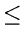i < n. A path is simple if all vertices on it are distinct, except possibly v1 and vn. The path has length n - 1 and connects v1 and vn.
• A graph is connected if every pair of vertices is connected.
• A subgraph G' = (V', E') of a graph G = (V, E) is a graph such that
1.
V'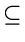V
2.
E' contains some edges (u, v) of E such that both u and vare in V'
If E' contains all edges (u, v)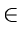E for which u, vV', the subgraph G' is called an induced subgraph.

• A connected component of a graph is an induced subgraph which is connected and which is not a proper subgraph of any other connected subgraph of G (i.e., maximal connected induced subgraph).
• A simple cycle is a simple path of length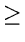3, that connects a vertex to itself.
• A graph is cyclic if it contains at least one (simple) cycle.
• A free tree is a connected, acyclic graph.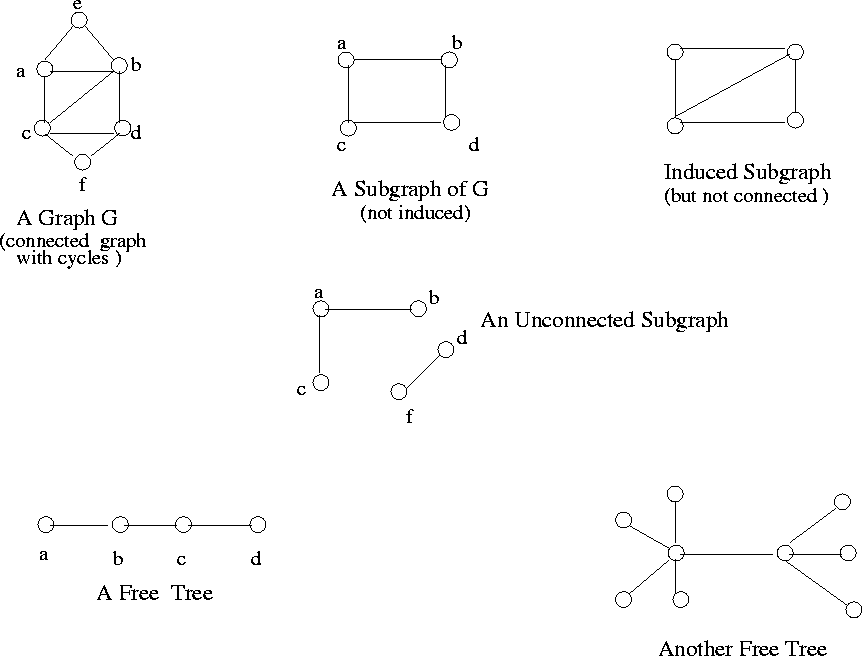• Observe that

1.
Every free tree with n vertices contains exactly n - 1 edges
2.
If we add any edge to a free tree, we get a cycle.
• See Figure 8.1 for several examples.Next: 8.2 Depth First and Breadth First Search Up: 8. Undirected Graphs Previous: 8. Undirected Graphs
eEL,CSA_Dept,IISc,Bangalore